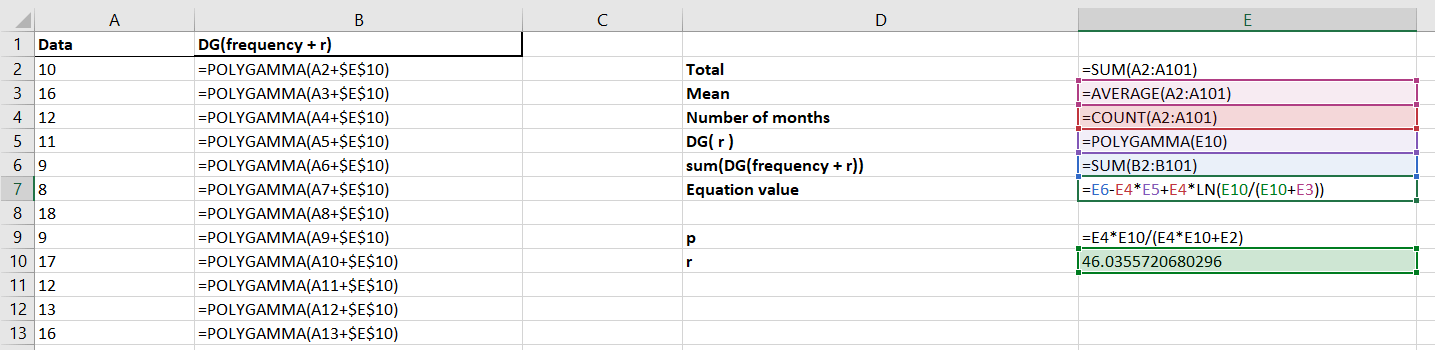Want to share your content on R-bloggers? click here if you have a blog, or here if you don't.

Recently I came across a situation where I had to use Microsoft Excel for a project. As somebody who has always used R for any statistical analysis, I may not have been entirely enthusiastic about the idea of leaving R behind for the world of Excel. But I figured it would be a good time to dip my toes in. In this blog post I discuss some of the things I found surprisingly difficult when learning Excel as an R user.

## Getting used to the lack of resources

One thing I particularly struggled with when using Excel was the difference in availability of free to use resources. In R there are thousands of open source packages available that either already do what you want or can assist you in getting to your goal. However, since Excel is not open source I found that, whilst resources sometimes already existed to do what I wanted to do, those resources were often pay-to-use. I ended up spending more time developing my own method for something that had already been done by somebody else. I’ve been spoiled by the open source nature of R and the availability of community-built packages that do a lot of my work for me.

Whether you want to start from scratch, or improve your skills, Jumping Rivers has a training course for you.

## Working with less common probability distributions

Before starting to work with Excel I think I was slightly over-optimistic about its capabilities for statistical analysis. I had hoped that there would be built in functions for working with most statistical distributions. Whilst there are built-in functions for some distributions, the availability of these functions is not very consistent. For example, for the Normal distribution there are built-in Excel functions for the PDF, CDF and inverse CDF. However, for the Negative Binomial distribution Excel only provides a function for the PDF and CDF of the distribution, and not for the inverse CDF. I found this inconsistency annoying as functions that I expected to exist, didn’t.

It is worth saying that there is a free to download resource pack available that does include functions for the inverse CDF of a Negative Binomial distribution, among other useful resources. It was the inconsistent availability of these functions (without the extra resources) that was a little annoying to me. It’s worth noting that base R also only includes functions for some of the most common probability distributions. However, at least R is consistent in which functions are available for the distributions.

## Increase in work required

As a consequence of the lack of available free resources, I found that I spent a lot of time and effort developing solutions to problems in Excel. That’s especially annoying when I know that it would only take one line of code in R.

As an example, when fitting a distribution in R, we can use the fitdistr() function from the {MASS} package. Let’s say we have some data that we think may follow a Negative Binomial distribution and we wish to estimate the parameters of the distribution from the data. If x is a vector of our data then we can estimate the parameters of the Negative Binomial distribution as follows:

library(MASS)
fitdistr(x, "negative binomial")


In contrast, in Excel this required an entire spreadsheet of work. The spreadsheet involved implementing maximum likelihood estimation of the distribution’s parameters by building formulae to numerically solve the following equation for r:

$$\left [ \sum_{i=1}^N \psi(x_i + r) \right ] – N\psi(r) + N \ln \left (\frac{r}{r + \sum_{i=1}^N x_i/N} \right ) = 0$$

and then using this to solve the following equation for p:

$$p = \frac{Nr}{Nr + \sum_{i=1}^N x_i}$$

where x1, …, xN are the data points and ψ() is the digamma function.

As I’m sure most people would agree – I’d rather not have to manually set up a spreadsheet to solve this equation (playing with digamma functions is not always fun). Especially when I know there’s a function in R that already does all of this for me behind the scenes.

Nevertheless, for the hundred data points contained in column A, the image below shows a screenshot of how this would work in Excel. On top of the formulae included, this also involved using the Excel Solver add-on to do the numerical estimation of r. Personally, I find this much more complicated than the single line in R!Many people use Excel every day without any problems, so many people must find working with it far easier than I did. However, I think I come from a relatively unusual place in having learnt to use R before I had a go at Excel. I was really surprised by how hard some things were to do that were really quick in R. However, I think this is down to having many years of practice with R, and very little practice at Excel. It’s true that for those without programming experience it may take a bit of time to figure out how to get started with R. But once you have picked up the basics it can be really quick to solve a lot of problems, and in my opinion, much easier!